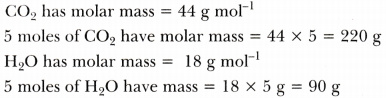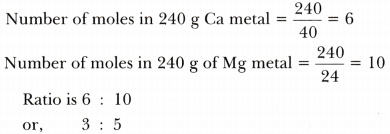# Verify by calculating that 5 moles of C02 and 5 moles of H20 do not have the same mass. 240 g of calcium and 240 g of magnesium elements have a mole ratio of 3 :5

Verify by calculating that

1. 5 moles of C02 and 5 moles of H20 do not have the same mass.
2. 240 g of calcium and 240 g of magnesium elements have a mole ratio of 3 :5.

1.2.